# ML Wiki

## Motivation

Suppose we have a binary classification problem:

• $y \in \{0, 1\}$ -
• 0 - negative class, connected with absence of smth (not spam)
• 1 - positive class, connected with presence of smth (spam)

### Linear Regression

We may try to use Linear Regression for that

• We fit a regression line
$h_{\theta}(x) = \theta^T x$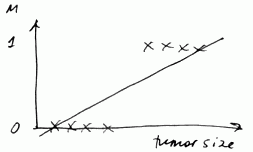• And we set a "threshold" at 0.5
• if $h_{\theta}(x) \geqslant 0.5$ - we predict 1
• if $h_{\theta}(x) < 0.5$ - we predict 0

What's wrong with this approach?

• Linear regression is susceptible to outliers - a single outlier can break the classificator
• $h_{\theta}(x)$ can be $> 1$ or $< 0$
• So linear regression is not a good idea

We need to develop something that outputs values from range $[0, 1]$ that we can treat as probabilities

## Logistic Regression

Logistic Regression - is a classification algorithm

### Hypothesis Representation

• we want - $0 \leqslant h_{\theta}(x) \leqslant 1$
• let $h_{\theta}(x) = g(\theta^T x)$
• where $g(z) = \cfrac{1}{1 + e^{-z}}$ - sigmoid (logistic) function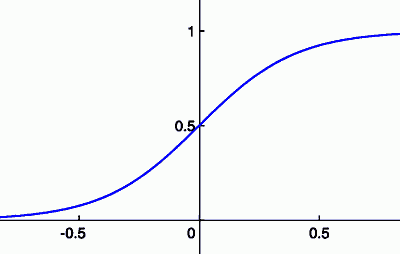it's always between 0 and 1

It inputs probability

• We interpret the output from $h_{\theta}(x)$as probability that $y = 1$ on input $x$, or
• $h_{\theta}(x) = P(y = 1 | x; \theta)$: probability that $y = 1$ given $x$ parametrized by $\theta$
• As $y = \{0, 1\}$, $P(y = 0| x; \theta) = 1 - P(y = 1 | x; \theta)$

For example, suppose $x = \left[\begin{matrix} x_0 \\ x_1 \end{matrix} \right] = \left[\begin{matrix} 1 \\ \text{tumor size} \end{matrix} \right]$

• if $h_{\theta}(x) = 0.7$, there's 70% chance of tumor being malignant

## Decision Boundary

Suppose we predict

• "$y = 1$" if $h_{\theta}(x) \geqslant 0.5$, and
• "$y = 0$" if $h_{\theta}(x) < 0.5$
• $g(z) \geqslant 0.5$ when $z \geqslant 0$• so $h_{\theta}(x) = g(\theta^T x) \geqslant 0.5$ when $\theta^T x \geqslant 0$

## Decision Boundary Line

• Assume that out model is $h_{\theta}(x) = g(\theta_0 + \theta_1 x_1 + \theta_2 x_2)$
• for $\theta = [-3, 1, 1]^T$
• $y = 1$ if $\theta^T x = -3 + x_1 + x_2 \geqslant 0$ (or $x_1 + x_2 \geqslant 3$)
• So we have the following decision boundary line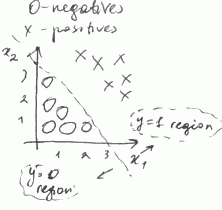• this line separates two regions: one with "$y = 1$" and another with "$y = 0$"

We may fit as complex expressions as we like

• Suppose we want non-linear decision boundary
• we fit polynomial expression:
$h_{\theta} = g(\theta_0 + \theta_1 x_1 + \theta_2 x_2 + \theta_3 x_1^2 + \theta_4 x_2^2)$
• Assume $\theta = [-1, 0, 0, 1, 1]^T$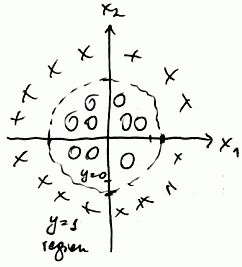• here we predict "$y = 1$" if $x_1^2 + x_2^2 \geqslant 1$

### Examples

Suppose we fit $h_{\theta}(x) = g(\theta_0 + \theta_1 x_1 + \theta_2 x_2)$

• with parameters $\theta_0 = -6$, $\theta_1 = 0$ and $\theta_2 = 1$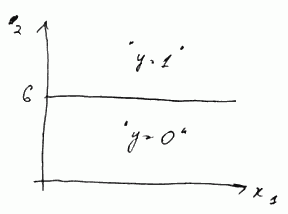the transition from negative to positive occur when x_2 goes from below 6 to above 6
• if parameters are $\theta_0 = 6$, $\theta_1 = -1$ and $\theta_2 = 0$,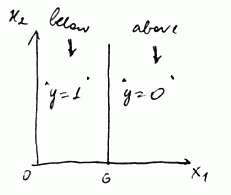the transition occurs when $x_1$ goes from above 6 to below 6 (note that $\theta_1 = -1$)

## Cost Function

We have:

• Training set $\{(x^{(i)}, y^{(i)})\}$ with $m$ examples
• each $x = [x_0, ..., x_n]^T \in \mathbb{R}^{n + 1}$ where $n$ is a number of features,
• all $x_0 = 1$
• and all $y \in \{0, 1\}$
• the model is $h_{\theta} = \cfrac{1}{1 - e^{-\theta^T x}}$
• How to choose parameters $\theta$?

### Non-Convex Cost Function

For Linear Regression the cost function was

• $J(\theta) = \cfrac{1}{m} \sum \text{cost}(h_{\theta}(x^{(i)}), y^{(i)})$
• where $\text{cost}(h_{\theta}(x), y) = \cfrac{1}{2} (h_{\theta}(x) - y)^2$

For logistic regression the problem with this approach is that with the sigmoid function g(z) it gives a non-convex function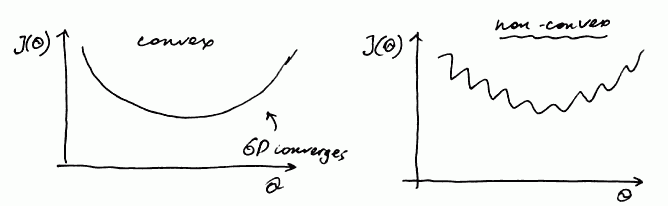• that is,
• if $J(\theta)$ is non-convex, it has many local optima, and Gradient Descent is not guaranteed to converge to a global optimum
• if $J(\theta)$ is convex, Gradient Descent always converges to a global optimum
• So we need a different cost function that is convex and GD will work on it

### Better Cost Function

We can use:

• $\text{cost}(h_{\theta}(x), y) = \left\{\begin{array}{l l} -\log(h_{\theta}(x)) & \text{ if } y = 1 \\ - \log(1 - h_{\theta}(x)) & \text{ if } y = 0 \end{array} \right.$
•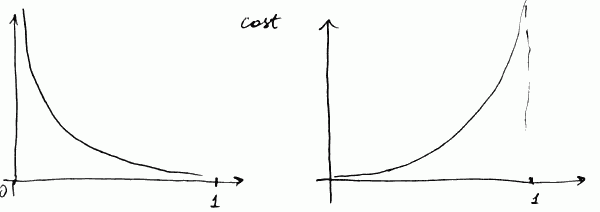• for the first equation (the left side)
• $\text{cost} = 0$ if $h_{\theta}(x) = 1$ (and $y = 1$)
• as $h_{\theta}(x) \to 0$, $\text{cost} \to \infty$
• it captures the intuition that if $h_{\theta}(x) = 0$, but $y = 1$, we will penalize by a very large cost
• same for the 2nd equation (the right side)
• $\text{cost} \to \infty$ as $h_{\theta}(x) \to 1$

Because $y \in \{0, 1\}$, we can rewrite the cost function as

• $\text{cost}(h_{\theta}(x), y) = -y \cdot \log(h_{\theta}(x)) - (1 - y) \cdot \log(1 - h_{\theta}(x))$
• and the total cost is $J(\theta) = \cfrac{1}{m} \sum \text{cost}(h_{\theta}(x^{(i)}), y^{(i)}) = - \cfrac{1}{m} \sum \left[ y \cdot \log(h_{\theta}(x)) + (1 - y) \cdot \log(1 - h_{\theta}(x)) \right]$

## Basic Algorithm

So the whole Logistic Regression algorithms is:

• find $\min_{\theta} J(\theta)$
• get this $\theta$
• for a new $x$ output $h_{\theta}(x) = g(\theta^T x)$
• using given threshold decide if $y = 1$ or $y = 0$

### Multi-Class Classification

For multi-class classification with Logistic Regression use One-vs-All Classification

### Regularization

Main Article: Regularization

To prevent overfitting we reduce magnitude of some features with Regularization

### Implementation

Matlab/Octave implementation: